Chemguide: Support for CIE A level Chemistry ``` ``` Learning outcome 25: Equilibria 25.2: Partition coefficients ``` ``` Learning outcomes 25.2.1, 25.2.2 and 25.2.3 This statement is about partition coefficents. Before you go on, you should find and read the statements in your copy of the syllabus. ``` ``` Partition If you have two immiscible liquids like ether and water, and shake them up in a separating funnel, they obviously form two layers. The ether is less dense than water, and so forms the top layer. Now suppose you shake up a mixture of ether and water containing a substance which is soluble in both of them. Let's suppose that the substance, X, is more soluble in ether than it is in water.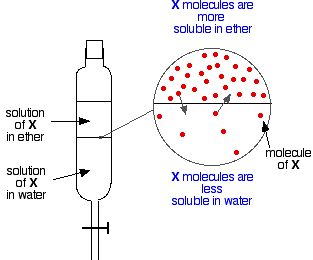Particles of X will cross the boundary between the two liquid layers, and you will soon get a dynamic equilibrium set up. For every particle which moves into the top layer, one will move back down into the bottom one. You could write an equation for this: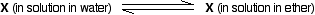. . . and like any other equilibrium, you can find an equilibrium constant: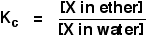This equilibrium constant is called the partition coefficient, and is often given the symbol Kpc. Like other equilibrium constants, partition coefficients are constant at a constant temperature, but they have some other restrictions as well. They only work properly for fairy dilute solutions, and the solute must be in the same chemical form in both solvents. It mustn't react, or ionise or associate (join together in dimers, for example). ``` ``` What governs the size of the partition coefficient? If you shake a small amount of bromine with water and tetrachloromethane, you get a very dark orange solution in the organic layer and a very pale orange solution in the aqueous layer. The bromine is obviously more soluble in the CCl4 than it is in water.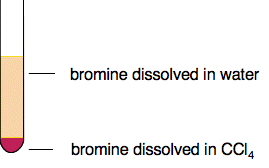Bromine is a simple case where the intermolecular attractions are just van der Waals dispersion forces. CCl4 also has van der Waals disperson forces as its only intermolecular force. Although there is polarity on each of the carbon-chlorine bonds, CCl4 as a whole is a non-polar molecule. Note:  If you aren't happy about that, you will find it discussed towards the bottom of the page about van der Waals forces. When bromine and CCl4 are mixed, the intermolecular forces being broken in each are replaced by very similar forces between the bromine and the CCl4. There isn't much energetic barrier to this happening. On the other hand, there are hydrogen bonds and permanent dipole-dipole attractions between water molecules as well as dispersion forces. These additional forces have to be broken when bromine molecules come between the water molecules, but are only replaced by simple dispersion forces between the bromine and the water. This isn't as energetically good. In general terms, the size of partition coefficients is governed by the relative solubility of the solute in the two solvents. That in turn is governed by the various strengths of the intermolecular attractions . . . in the solute, in both of the two solvents, between solute molecules and each of the solvents. The case we have looked at above is typical of the thinking that you would have to do if you were asked to comment on the size of a partition coefficient in any other case you might come across. ``` ``` Partition coefficient calculations A note on units Notice that the partition coefficient is a simple ratio of two concentrations. It doesn't matter what concentration units you use - as long as you use the same ones top and bottom. You could use mol dm-3, but more often you use g cm-3 - grams per cubic centimetre. Technically, the square brackets can only be used for concentration in mol dm-3, but the Application Support Booklet and CIE's mark schemes both use it for other units as well. I'm not prepared to do that, and so shall use the term "concentration of X" rather than [X] where non-standard concentration units are used. ``` ``` Calculating a partition coefficient When a solution of 1.00 g of X in 100 cm3 of water was shaken with 10 cm3 of ether, 0.80 g of X was transferred to the ether layer. Calculate the partition coefficient of X between ether and water. If you are asked to calculate a partition coefficient between two solvents, the concentration of the first solvent mentioned goes on top of the Kpc expression. So in this case: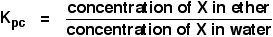You have enough information to calculate both concentrations in g cm-3.concentration of X in ether = 0.80/10 g cm-3 If 0.80 g were transferred to the ether, 1.00 - 0.80 g = 0.20 g were left in the water.concentration of X in water = 0.20/100 g cm-3 So: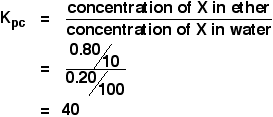Obviously, you could work out the concentrations in ether and in water as actual numbers before you put them into the expression. Do it however you feel most comfortable. Partition coefficients like this don't have units - the units cancel out because they are the same top and bottom. ``` ``` Calculations involving partition coefficients A basic example We will use the same case as before - the same solvents, the same X and the same partition coefficient we have just calculated. This time we will work out how much would have been extracted into the ether layer if we had shaken the original solution of 1.00 g of X in 100 cm3 of water with just 5 cm3 of ether. We are trying to work out the mass of X extracted. Let's call that m. Now work out an expression for the concentration of the solution of X in ether.concentration of X in ether = m/5 g cm-3 What about the water? There will be (1.00 - m) g of X left in the water. So:concentration of X in water = (1.00 - m)/100 g cm-3 Now you can put all this into the partition coefficient expression. Remember that we have already calculated the partition coefficient of X between ether and water as 40.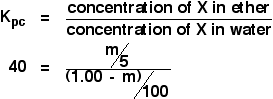You are then just faced with a simple, but slightly tedious, bit of algebra: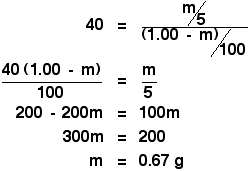``` ``` Making it more tedious! In the original calculation to find the partition coefficient, you were told that if you shook the original solution of 1.00 g of X in 100 cm3 of water with 10 cm3 of ether, you extracted 0.80 g of X. Shaking it with 5 cm3 of ether, we have just worked out that you would extract 0.67 g of X. That would leave 0.33 g of X behind in the 100 cm3 of water. Suppose you had carefully kept this solution, and then shook it with a second fresh 5 cm3 of ether. How much of X would you extract in total by using the ether as two separate lots of 5 cm3 instead of the 10 cm3 in one go? Let's call the mass of X extracted by the second lot of ether n - so that we don't get confused. Work out an expression for the concentration of the solution of X in ether.concentration of X in ether = n/5 g cm-3 What about the water? There will be (0.33 - n) g of X left in the water after the second extraction. So:concentration of X in water = (0.33 - n)/100 g cm-3 Now you can put all this into the partition coefficient expression for X between ether and water as before.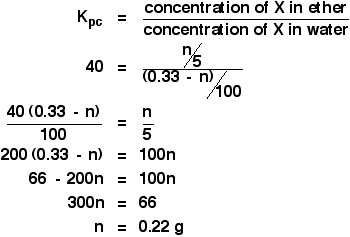That means that if you were to combine the two 5 cm3 lots of ether, you would have extracted a total of 0.67 + 0.22 g of X. That is 0.89 g. You were originally told that if you had only done this once, using the ether as a single lot of 10 cm3, you would only have extracted 0.80 g. You get a more efficient extraction by splitting your solvent up into smaller volumes as above. You use this sort of technique during the preparation of some organic compounds. You extract what you are trying to make from some messy solution in water so that it ends up in an organic solvent. You then remove the solvent by careful distillation. ``` ``` CIE asked a question similar to this in June 2009 paper 4 Q8. You had to calculate a value for the partition coefficient, and then use it in a two step extraction exactly as above. The calculation was worth 4 marks. This may be tedious, but you can't afford not to be able to do it. Note:  If you have a copy of my calculations book, I would suggest that you don't read about these multi-step calculations in the book. I use a much more efficient, and less tedious, way of doing them, but CIE doesn't use it. You risk confusing yourself. ``` ``` Go to the Section 25 Menu . . . To return to the list of learning outcomes in Section 25 Go to the CIE Main Menu . . . To return to the list of all the CIE sections Go to Chemguide Main Menu . . . This will take you to the main part of Chemguide. © Jim Clark 2020